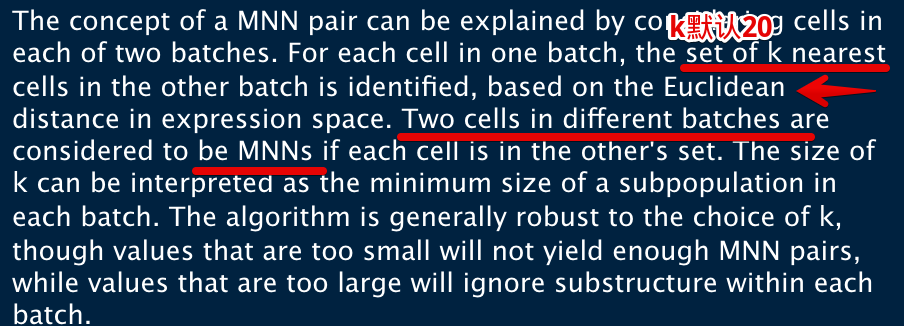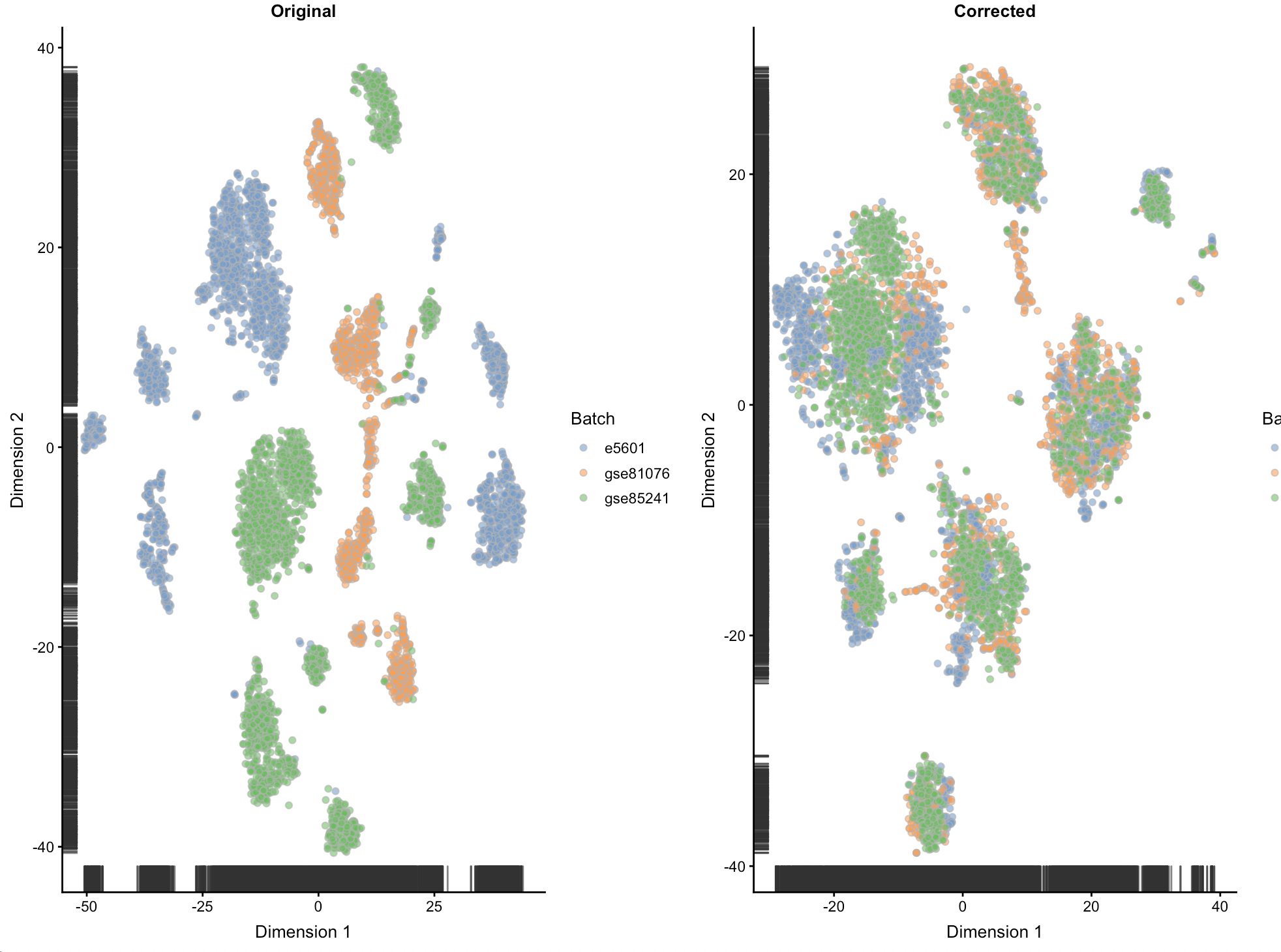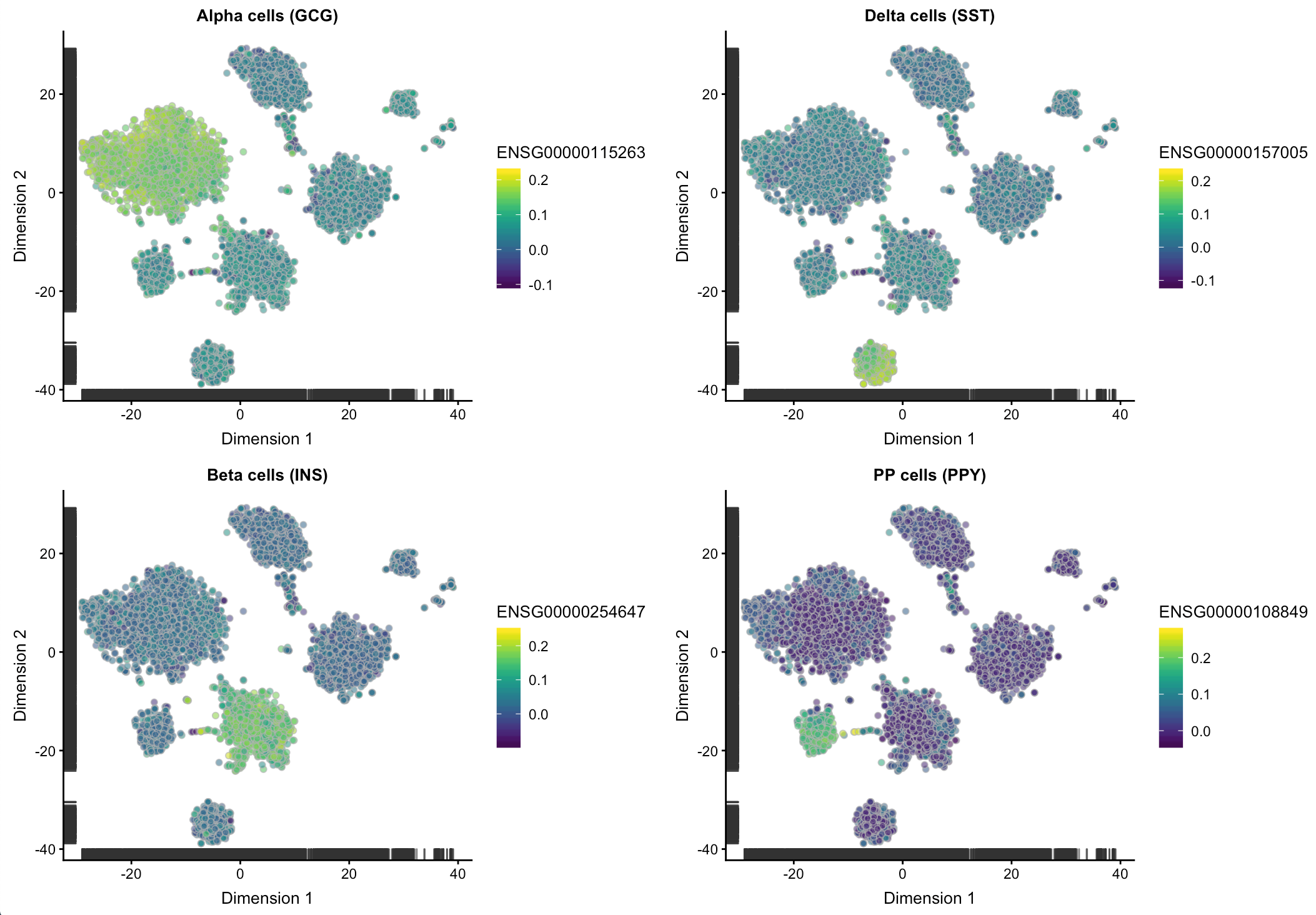# 单细胞转录组数据校正批次效应实战（下）

### 三组不同数据的混合

#### 整合ID

```rm(list = ls())
options(stringsAsFactors = F)
# 选择前1000基因
top.e5601 <- rownames(dec.e5601)[seq_len(1000)]
top.gse85241 <- rownames(dec.gse85241)[seq_len(1000)]
top.gse81076 <- rownames(dec.gse81076)[seq_len(1000)]
# https://www.r-bloggers.com/intersect-for-multiple-vectors-in-r/
chosen <- Reduce(intersect, list(top.e5601, top.gse85241, top.gse81076))

# 添加gene symbol
symb <- mapIds(org.Hs.eg.db, keys=chosen, keytype="ENSEMBL", column="SYMBOL")
> DataFrame(ID=chosen, Symbol=symb)
DataFrame with 353 rows and 2 columns
ID      Symbol
<character> <character>
1   ENSG00000115263         GCG
2   ENSG00000118271         TTR
3   ENSG00000089199        CHGB
4   ENSG00000169903      TM4SF4
5   ENSG00000166922        SCG5
...             ...         ...
349 ENSG00000087086         FTL
351 ENSG00000162545     CAMK2N1
352 ENSG00000170348      TMED10
353 ENSG00000251562      MALAT1
```

```in.all <- Reduce(intersect, list(rownames(dec.e5601),
rownames(dec.gse85241), rownames(dec.gse81076)))

# 设置权重weighted=FALSE ，认为每个batch的贡献一致
combined <- combineVar(dec.e5601[in.all,], dec.gse85241[in.all,],
dec.gse81076[in.all,], weighted=FALSE)
```

#### 进行基于MNN的校正

Haghverdi et al. (2018)：MNN pairs represent cells from the same biological state prior to the application of a batch effect```original <- list(logcounts(sce.e5601)[chosen,],
logcounts(sce.gse81076)[chosen,],
logcounts(sce.gse85241)[chosen,])
corrected <- do.call(mnnCorrect, c(original, list(k=20, sigma=0.1)))
> str(corrected\$corrected)
List of 3
\$ : num [1:353, 1:2285] 0.127 0.137 0.121 0 0.113 ...
..- attr(*, "dimnames")=List of 2
.. ..\$ : chr [1:353] "ENSG00000115263" "ENSG00000118271" "ENSG00000089199" "ENSG00000169903" ...
.. ..\$ : chr [1:2285] "HP1502401_J13" "HP1502401_H13" "HP1502401_J14" "HP1502401_B14" ...
\$ : num [1:353, 1:1292] -0.01724 -0.0062 0.01149 0.00689 0.01272 ...
\$ : num [1:353, 1:2346] 0.142 0.138 0.132 0.109 0.11 ...
```

• `k` 表示在定义MNN pair时，设置几个最近的邻居(nearest neighbours )，表示每个batch中每种细胞类型或状态出现的最低频率。增大这个数字，会通过增加MNN pair数量来增加矫正的精度，但是需要允许在不同细胞类型之间形成MNN pair，这一操作又会降低准确性，所以需要权衡这个数字增大k值，会提高precision，降低accuracy
• `sigma` 表示在计算批次效应时如何定义MNN pair之间共享的信息量。较大的值会共享更多信息，就像对同一批次的所有细胞都进行校正；较小的值允许跨细胞类型进行校正，可能会更准确，但会降低精度。默认值为1，比较保守的一个设定，校正不会太多，但多数情况选择小一点的值会更合适减小sigma，会增加accuracy，降低precision

这里很有必要说明两个英语词汇：

• Accuracy refers to the closeness of a measured value to a standard or known value. 和标准值比是否"准确"
• Precision refers to the closeness of two or more measurements to each other. 相互之间比是否"精确"
• 另外，提供的original list中各个batch的顺序是很重要的，因为是将第一个batch作为校正的参考坐标系统。一般推荐设置批次效应最大或异质性最强的批次作为对照，可以保证参考批次与其他校正批次之间有充足的MNN pair

#### 检验校正的作用

```# omat是原始矩阵，mat是校正后的
omat <- do.call(cbind, original)
mat <- do.call(cbind, corrected\$corrected)
# 将mat列名去掉
colnames(mat) <- NULL
sce <- SingleCellExperiment(list(original=omat, corrected=mat))
# 用lapply对三个列表进行循环操作，求列数，为了给rep设置一个重复值
colData(sce)\$Batch <- rep(c("e5601", "gse81076", "gse85241"),
lapply(corrected\$corrected, ncol))

> sce
class: SingleCellExperiment
dim: 353 5923
assays(2): original corrected
rownames(353): ENSG00000115263 ENSG00000118271 ... ENSG00000170348
ENSG00000251562
rowData names(0):
colnames(5923): HP1502401_J13 HP1502401_H13 ... D30.8_93 D30.8_94
colData names(1): Batch
reducedDimNames(0):
spikeNames(0):
```
##### 做个t-sne图来看看

```osce <- runTSNE(sce, exprs_values="original", set.seed=100)
ot <- plotTSNE(osce, colour_by="Batch") + ggtitle("Original")
csce <- runTSNE(sce, exprs_values="corrected", set.seed=100)
ct <- plotTSNE(csce, colour_by="Batch") + ggtitle("Corrected")
multiplot(ot, ct, cols=2)
``````ct.gcg <- plotTSNE(csce, by_exprs_values="corrected",
colour_by="ENSG00000115263") + ggtitle("Alpha cells (GCG)")
ct.ins <- plotTSNE(csce, by_exprs_values="corrected",
colour_by="ENSG00000254647") + ggtitle("Beta cells (INS)")
ct.sst <- plotTSNE(csce, by_exprs_values="corrected",
colour_by="ENSG00000157005") + ggtitle("Delta cells (SST)")
ct.ppy <- plotTSNE(csce, by_exprs_values="corrected",
colour_by="ENSG00000108849") + ggtitle("PP cells (PPY)")
multiplot(ct.gcg, ct.ins, ct.sst, ct.ppy, cols=2)
```• 本文由 发表
• 转载请务必保留本文链接：https://www.plob.org/article/20834.html